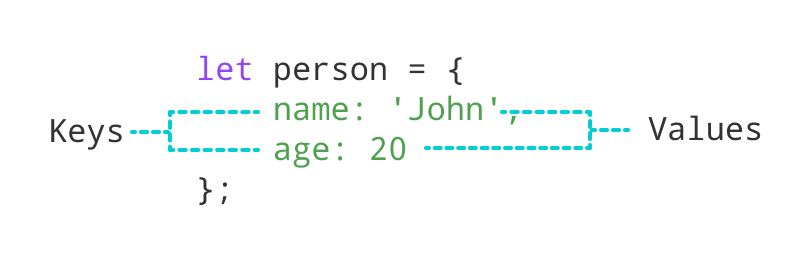# JavaScript Objects

In this tutorial, you will learn about JavaScript objects with the help of examples.

In the JavaScript data types tutorial, you learned about 7 different primitive data types. And here, you are going to learn about the eighth data-type (JavaScript object).

JavaScript object is a non-primitive data-type that allows you to store multiple collections of data.

Note: If you are familiar with other programming languages, JavaScript objects are a bit different. You do not need to create classes in order to create objects.

Here is an example of a JavaScript object.

``````// object
const student = {
firstName: 'ram',
class: 10
};``````

Here, `student` is an object that stores values such as strings and numbers.

## JavaScript Object Declaration

The syntax to declare an object is:

``````const object_name = {
key1: value1,
key2: value2
}``````

Here, an object `object_name` is defined. Each member of an object is a key: value pair separated by commas and enclosed in curly braces `{}`.

For example,

``````// object creation
const person = {
name: 'John',
age: 20
};
console.log(typeof person); // object``````

You can also define an object in a single line.

``const person = { name: 'John', age: 20 };``

In the above example, `name` and `age` are keys, and `John` and `20` are values respectively.

There are other ways to declare an object in JavaScript. To learn more, visit Different Ways to Declare JavaScript Objects.

## JavaScript Object Properties

In JavaScript, "key: value" pairs are called properties. For example,

``````let person = {
name: 'John',
age: 20
};``````

Here, `name: 'John'` and `age: 20` are properties.JavaScript object properties

## Accessing Object Properties

You can access the value of a property by using its key.

### 1. Using dot Notation

Here's the syntax of the dot notation.

``objectName.key``

For example,

``````const person = {
name: 'John',
age: 20,
};

// accessing property
console.log(person.name); // John``````

### 2. Using bracket Notation

Here is the syntax of the bracket notation.

``objectName["propertyName"]``

For example,

``````const person = {
name: 'John',
age: 20,
};

// accessing property
console.log(person["name"]); // John``````

## JavaScript Nested Objects

An object can also contain another object. For example,

``````// nested object
const student = {
name: 'John',
age: 20,
marks: {
science: 70,
math: 75
}
}

// accessing property of student object
console.log(student.marks); // {science: 70, math: 75}

// accessing property of marks object
console.log(student.marks.science); // 70``````

In the above example, an object `student` contains an object value in the `marks` property.

## JavaScript Object Methods

In JavaScript, an object can also contain a function. For example,

``````const person = {
name: 'Sam',
age: 30,
// using function as a value
greet: function() { console.log('hello') }
}

person.greet(); // hello``````

Here, a function is used as a value for the greet key. That's why we need to use `person.greet()` instead of `person.greet` to call the function inside the object.

A JavaScript method is a property containing a function declaration. In the next tutorial, you will learn about JavaScript Methods in detail.

Did you find this article helpful?